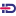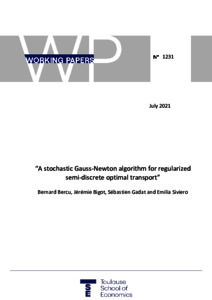Increase the visibility of your scientific production by authorizing the export of your publications to HAL!

# A Stochastic Gauss-Newton Algorithm for Regularized Semi-discrete Optimal Transport

Gadat, Sébastien, Bercu, Bernard, Bigot, Jérémieand Siviero, Emilia (2021) A Stochastic Gauss-Newton Algorithm for Regularized Semi-discrete Optimal Transport. TSE Working Paper, n. 21.1231, Toulouse, FrancePreview
Text
Official URL : http://tse-fr.eu/pub/125790

## Abstract

We introduce a new second order stochastic algorithm to estimate the entropically regularized optimal transport cost between two probability measures. The source measure can be arbitrary chosen, either absolutely continuous or discrete, while the target measure is assumed to be discrete. To solve the semi-dual formulation of such a regularized and semi-discrete optimal transportation problem, we propose to consider a stochastic Gauss-Newton algorithm that uses a sequence of data sampled from the source measure. This algorithm is shown to be adaptive to the geometry of the underlying convex optimization problem with no important hyperparameter to be accurately tuned. We establish the almost sure convergence and the asymptotic normality of various estimators of interest that are constructed from this stochastic Gauss-Newton algorithm. We also analyze their non-asymptotic rates of convergence for the expected quadratic risk in the absence of strong convexity of the underlying objective function. The results of numerical experiments from simulated data are also reported to illustrate the nite sample properties of this Gauss-Newton algorithm for stochastic regularized optimal transport, and to show its advantages over the use of the stochastic gradient descent, stochastic Newton and ADAM algorithms.

Item Type: Monograph (Working Paper) English July 2021 Toulouse, France Stochastic optimization, Stochastic Gauss-Newton algorithm, Optimal transport, Entropic regularization, Convergence of random variables. B- ECONOMIE ET FINANCE TSE-R (Toulouse) Université Toulouse 1 Capitole UT1 28 Jul 2021 12:43 01 Sep 2021 09:00 oai:tse-fr.eu:125790 http://publications.ut-capitole.fr/id/eprint/43699View Item